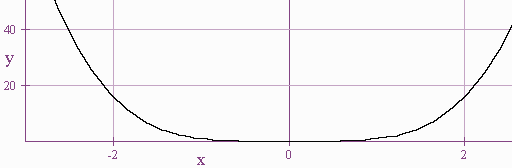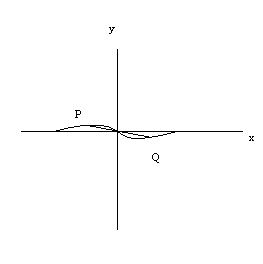$$\newcommand{\id}{\mathrm{id}}$$ $$\newcommand{\Span}{\mathrm{span}}$$ $$\newcommand{\kernel}{\mathrm{null}\,}$$ $$\newcommand{\range}{\mathrm{range}\,}$$ $$\newcommand{\RealPart}{\mathrm{Re}}$$ $$\newcommand{\ImaginaryPart}{\mathrm{Im}}$$ $$\newcommand{\Argument}{\mathrm{Arg}}$$ $$\newcommand{\norm}{\| #1 \|}$$ $$\newcommand{\inner}{\langle #1, #2 \rangle}$$ $$\newcommand{\Span}{\mathrm{span}}$$

# 1.2: Graphs and Symmetry

$$\newcommand{\vecs}{\overset { \rightharpoonup} {\mathbf{#1}} }$$ $$\newcommand{\vecd}{\overset{-\!-\!\rightharpoonup}{\vphantom{a}\smash {#1}}}$$$$\newcommand{\id}{\mathrm{id}}$$ $$\newcommand{\Span}{\mathrm{span}}$$ $$\newcommand{\kernel}{\mathrm{null}\,}$$ $$\newcommand{\range}{\mathrm{range}\,}$$ $$\newcommand{\RealPart}{\mathrm{Re}}$$ $$\newcommand{\ImaginaryPart}{\mathrm{Im}}$$ $$\newcommand{\Argument}{\mathrm{Arg}}$$ $$\newcommand{\norm}{\| #1 \|}$$ $$\newcommand{\inner}{\langle #1, #2 \rangle}$$ $$\newcommand{\Span}{\mathrm{span}}$$ $$\newcommand{\id}{\mathrm{id}}$$ $$\newcommand{\Span}{\mathrm{span}}$$ $$\newcommand{\kernel}{\mathrm{null}\,}$$ $$\newcommand{\range}{\mathrm{range}\,}$$ $$\newcommand{\RealPart}{\mathrm{Re}}$$ $$\newcommand{\ImaginaryPart}{\mathrm{Im}}$$ $$\newcommand{\Argument}{\mathrm{Arg}}$$ $$\newcommand{\norm}{\| #1 \|}$$ $$\newcommand{\inner}{\langle #1, #2 \rangle}$$ $$\newcommand{\Span}{\mathrm{span}}$$

## Symmetry (Geometry)

Definition: Symmetric with respect to the y-axis

We say that a graph is symmetric with respect to the y-axis if for every point $$(a,b)$$ on the graph, there is also a point $$(-a,b)$$ on the graph; hence $f(x,y) = f(-x,y).$

Visually we have that the y-axis acts as a mirror for the graph. We will demonstrate several functions to test for symmetry graphically using the graphing calculator.Definition: Symmetric with respect to the x-axis

We say that a graph is symmetric with respect to the x axis if for every point $$(a,b)$$ on the graph, there is also a point $$(a,-b)$$ on the graph; hence $f(x,y) = f(x,-y).$

Visually we have that the x-axis acts as a mirror for the graph. We will demonstrate several functions to test for symmetry graphically using the graphing calculator.

Definition: Symmetry with respect to Origin

We say that a graph is symmetric with respect to the origin if for every point $$(a,b)$$ on the graph, there is also a point $$(-a,-b)$$ on the graph; hence $f(x,y) = f(-x,-y).$

Visually we have that given a point $$P$$ on the graph if we draw a line segment $$PQ$$ through $$P$$ and the origin such that the origin is the midpoint of $$PQ$$, then $$Q$$ is also on the graph.We will use the graphing calculator to test for all three symmetries.

## x-axis Symmetry

To test algebraically if a graph is symmetric with respect the x-axis, we replace all the $$y$$'s with $$-y$$ and see if we get an equivalent expression.

Example $$\PageIndex{1}$$

For

$x - 2y = 5$

we replace with

$x - 2(-y) = 5.$

Simplifying we get

$x+2y=5.$

Which is not equivalent to the original expression. So

$x - 2y = 5$

Is not symmetric with respect to the x-axis.

Example $$\PageIndex{2}$$

For

$x^3 - y^2 = 2$

we replace with

$x^3 - (-y)^2 = 2$

which is equivalent to the original expression, so that

$x^3 - y^2 = 2$

is symmetric with respect to the x-axis.

## y-axis Symmetry

To test algebraically if a graph is symmetric with respect to the y axis, we replace all the x's with -x and see if we get an equivalent expression.

Example $$\PageIndex{3}$$

For

$y = x^2$

we replace with

$y = (-x)^2 = x^2$

so that

$y = x^2$

is symmetric with respect to the y-axis.

Example $$\PageIndex{4}$$

For

$y = x^3$

we replace with

$y = (-x)^3 = - x^3$

so that

$y = x^3$

is not symmetric with respect to the y-axis.

## Origin Symmetry

To test algebraically if a graph is symmetric with respect to the origin we replace both $$x$$ and $$y$$ with $$-x$$ and $$-y$$ and see if the result is equivalent to the original expression.

Example $$\PageIndex{5}$$

For

$y = x^3$

we replace with

$(-y) = (-x)^3$

so that

$-y = -x^3 \text{ or } y=x^3.$

Hence

$y = x^3$

is symmetric with respect to the origin.

## Intercepts

We define the x intercepts as the points on the graph where the graph crosses the x axis. If a point is on the x axis, then the y coordinate of the point is 0. Hence to find the x intercepts, we set $$y = 0$$ and solve.

Example $$\PageIndex{6}$$: x intercepts

Find the x intercepts of

$y = x^2+ x - 2$

Solution

We set $$y = 0$$ so that

$0 = x^2+ x - 2 = (x + 2)(x - 1)$

Hence that x intercepts are at $$(-2,0)$$ and $$(1,0)$$

We define the y intercepts of a graph to be the points where the graph crosses the y-axis. At these points the $$x$$ coordinate is 0 hence to find the y intercepts we set $$x = 0$$ and find $$y$$.

Example $$\PageIndex{7}$$: y intercepts

Find the y intercepts of

$y = x^2+ x - 2$

Solution

We set $$x = 0$$ to get:

$y = 0 + 0 - 2 = -2.$

Hence the y intercept is at $$(0,-2)$$.